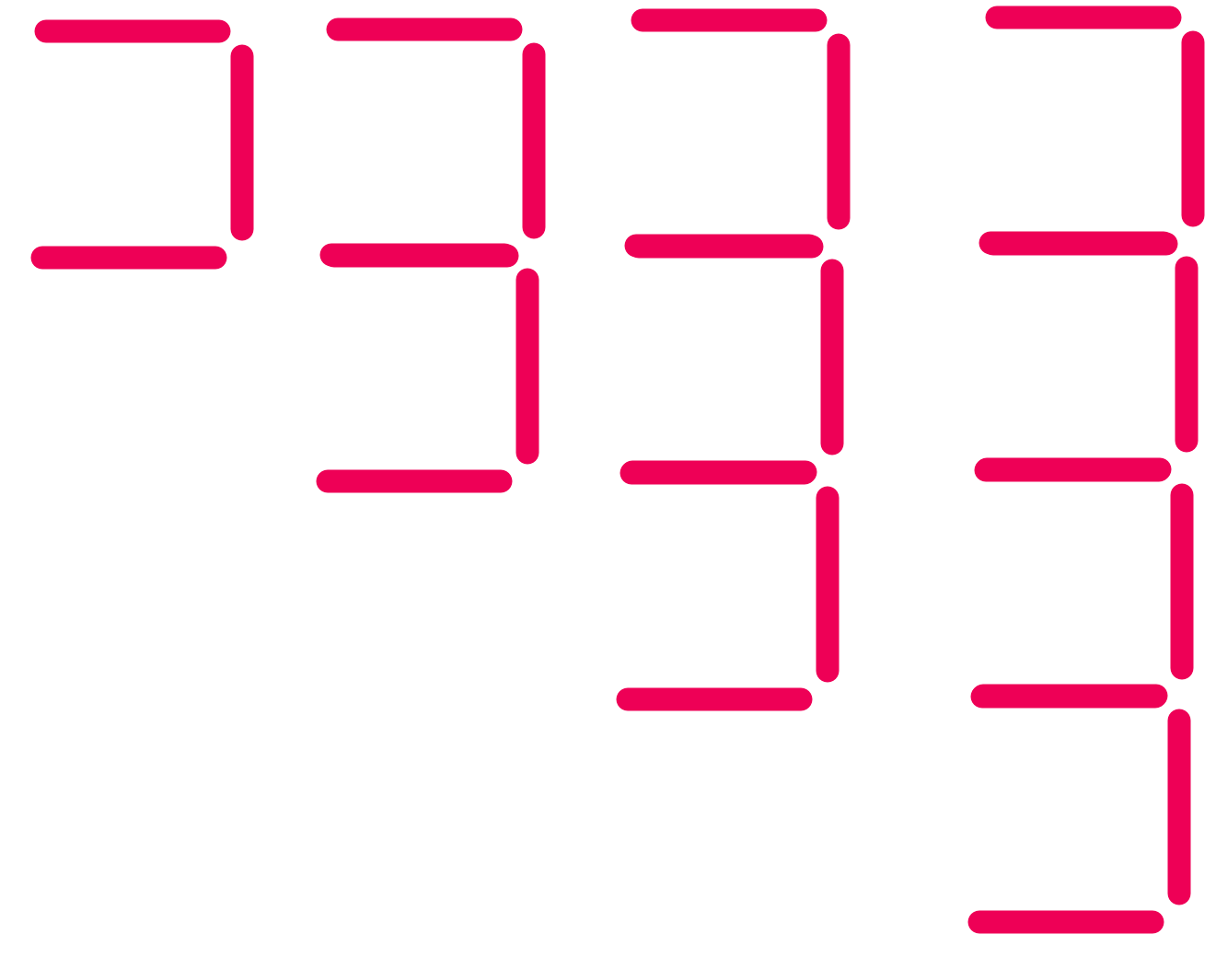Generating Terms

## Generating Terms

A sequence is a list of numbers (or a pattern) that are related to each other by a rule. The next number or pattern in the list can be worked out from the previous number or pattern using this rule.

Each number in the list is called a term. The first number is the first term, the second number is the second term, and so on.

The rule that links the terms is called the term-to-term rule.

To work out the next term in a sequence, work out what the rule is.

After a term-to-term rule has been worked out, always compare it against another pair of terms to make sure that it is correct.

## Example 1

A term-to-term rule is given as multiply the last term by 2 and subtract 3.

What are the next two terms in the sequence, if the first term is 5?

 Second term: (5) x 2 - 3 = 7 Third term: (7) x 2 - 3 = 11

## Example 2

A sequence is made up from a set of lines.

How many lines will there be in the 5th and 6th terms of the sequence?The first term has 3 lines; the second term has 5 lines. The term-to-term rule is add two lines. Check the next pair of terms: this is also add two lines. Use a table to work out the answer.

 Term 1 2 3 4 5 6 Number of Sticks 3 5 7 9 11 13